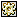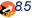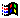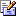Contents Articles Behaviors Books Director News Director Web Sites FAQ Games Mailing Lists News Groups Project Examples Reviews Software Tools Useful Web Sites Utilities Xtras

 Don't miss these#Vector Shape - Create Star

 Compatibilities:This item has not yet been rated

Author: Chunick (website)

Pass this custom handler the width, height and number of points to create a star shape. You can pass the other two optional parameters: sharpness and rotate. The sharpness is a value between -1 and 1 and determines how "pointy" the star is. The rotation affects the inside points only.

 on createStar (width, height, points, sharpness, rotate)      vertList = []      if voidP(sharpness) then     Case points of       5:         sharpness = .38       6:         sharpness = .58                otherwise         sharpness = .5     end Case   end if      points = integer(points) * 2      if voidP(rotate) then rotate = 0      a = max(width, height) / 2.0   b = min(width, height) / 2.0   a2 = a * sharpness   b2 = b * sharpness      rad = (360 / float(points)) * (pi / 180)   radOffset = rad + (90 * (pi / 180))   rotate = rotate * (pi / 180) --rad * (rotate * (pi / 180) / (360 * (pi / 180)))      repeat with i = 1 to points     t = (i * rad) - radOffset     if i mod 2 = 0 then       x = a2 * cos(t + rotate)       y = b2 * sin(t + rotate)     else       x = a * cos(t)       y = b * sin(t)     end if     vertList.add([#vertex: point(x, y)])   end repeat      return vertList    end

 Contact MMI 36 South Court Sq Suite 300 Newnan, GA 30263 USASend e-mail